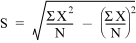# 16.20 - Using a C Structure to Access the Storage Area - Advanced SQL Engine - Teradata Database

## Teradata Vantage™ - SQL External Routine Programming

Product
Release Number
16.20
Release Date
April 2020
Content Type
Programming Reference
Publication ID
B035-1147-162K
Language
English (United States)

The type of intermediate results that an aggregate function needs to save depends on the type of calculation that the function performs.

Consider a standard deviation function STD_DEV(x) that uses the following equation:Based on the calculation, the function needs to store the following:
• N
• sum(X 2)
• sum(X)

The function can declare a C structure with components that match the necessary intermediate values:

```typedef struct agr_storage {
FLOAT n;
FLOAT x_sq;
FLOAT x_sum;
} AGR_Storage;```

The function can then define a pointer to AGR_Storage that points to the FNC_Context_t.interim1 function argument.

Suppose the function declaration uses the following parameters:

```void STD_DEV ( FNC_Phase     phase,
FNC_Context_t *fctx,
FLOAT         *x,
FLOAT         *result,
char          sqlstate )
{
...
}```

The following statement defines a pointer to AGR_Storage named s1 that points to the FNC_Context_t.interim1 function argument:

`AGR_Storage *s1 = fctx->interim1;`

The function can then easily access the storage area using the pointer to AGR_Storage. For example:

```s1->n     = 0;
s1->x_sq  = 0;
s1->x_sum = 0;```